Next: Joule-Thompson Throttling Up: Classical Thermodynamics Previous: Free Expansion of Gas

# Van der Waals Gas

Consider a non-ideal gas whose equation of state takes the form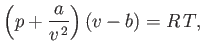(6.156)

where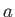and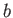are positive constants. (See Section 8.11.) Such a gas is known as a van der Waals gas. The previous approximate equation of state attempts to take into account the existence of long-range attractive forces between molecules in real gases, as well as the finite volume occupied by the molecules themselves. The attractive forces gives rise to a slight compression of the gas (relative to an ideal gas)--the term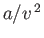represents this additional positive pressure. The parameteris the volume occupied by a mole of gas molecules. Thus,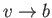as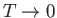. Of course, in the limit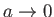and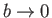, the van der Waals equation of state reduces to the ideal gas equation of state.

Table 6.2: Critical pressures and temperatures, and derived van der Waals parameters, for common gases.
 Gas Symbol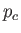(bar)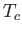(K)(SI)(SI) Helium He 2.27 5.19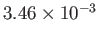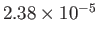Hydrogen H 13.0 33.2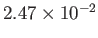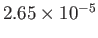Nitrogen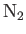33.9 126.2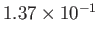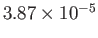Oxygen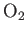50.5 154.6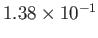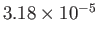The van der Waals equation of state can be written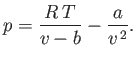(6.157)

At fixed temperature, the previous equation yields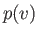curves that exhibit a maximum and a minimum at two points where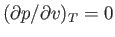. At a particular temperature, the maximum and minimum coalesce into a single inflection point where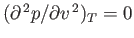, in addition to. This point is called the critical point, and its temperature, pressure, and molar volume are denoted,, and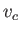, respectively. It is readily demonstrated that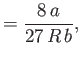(6.158)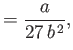(6.159)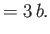(6.160)

(See Exercise 13.) The critical temperature,, and the critical pressure,, of a substance are easily determined experimentally, because they turn out to be the maximum temperature and pressure, respectively, at which distinct liquid and gas phases exist. (See Section 9.10.) This allows a determination of the constantsandin the van der Waals equation of state. In fact,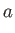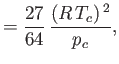(6.161)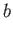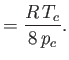(6.162)

Table 6.2 shows the experimentally measured critical pressures and temperatures, as well as the derived van der Waal parameters, for some common gases.

Let us calculate the Joule coefficient for a van der Waals gas. It follows from Equation (6.156) that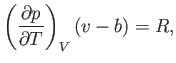(6.163)

which implies that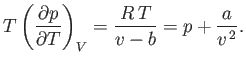(6.164)

Substitution into Equation (6.155) gives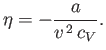(6.165)

Thus, the Joule coefficient for a van der Waals gas is negative. This implies that the temperature of the gas always decreases as it undergoes free expansion. Of course, this temperature decrease is a consequence of the work done in overcoming the inter-molecular attractive forces. Over a relatively small temperature range,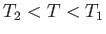, any possible temperature dependence of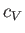is negligibly small. Thus,can be regarded as essentially constant, and Equations (6.149) and (6.165) yield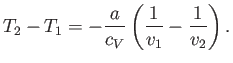(6.166)

For an expansion, where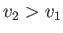, this equation confirms that. In other words, the temperature of non-ideal gas that undergoes free expansion is reduced.

In principle, it appears that the free expansion of a gas could provide a method of cooling the gas to low temperatures. In practice, a substantial difficulty arises because of the appreciable heat capacity,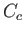, of the container. Because the container's internal energy changes by an amount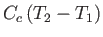, the molar heat capacity,, in Equation (6.166), must be replaced by the total molar heat capacity,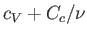. Given that the heat capacity of the container is generally much greater than that of the gas (i.e.,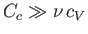), it follows that the actual temperature reduction is much smaller than that predicted by Equation (6.166).Next: Joule-Thompson Throttling Up: Classical Thermodynamics Previous: Free Expansion of Gas
Richard Fitzpatrick 2016-01-25